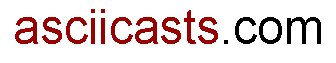#ASCIIcasts

## 14: Performing Calculations On Models

(view original Railscast)

ActiveRecord provides many different methods for performing calculations on models. To demonstrate this we’ll use a `Task` model that has an integer field called `priority` and look at some of those methods in `script/console`. For each method the SQL generated by Rails is shown afterwards.

We can find the priority of the first task like this:

``` >> Task.first.priority => 3 ``` ` SELECT * FROM "tasks" LIMIT 1`

What if we want the sum of the priority of all of the tasks?

``` >> Task.sum(:priority) => 12 ``` `SELECT sum("tasks".priority) AS sum_priority FROM "tasks"`

We can even pass conditions:

``` >> Task.sum(:priority, :conditions => {:completed => false }) => 2 ``` `SELECT sum("tasks".priority) AS sum_priority FROM "tasks" WHERE (completed="f")`

As well as `sum` there are other methods, such as `minimum`, `maximum` and `average`:

``` >> Task.minimum(:priority) => 2 ``` `SELECT min("tasks".priority) AS min_priority FROM "tasks"` ``` >> Task.maximum(:priority) => 5 ``` `SELECT max("tasks".priority) AS max_priority FROM "tasks"` ``` >> Task.average(:priority).to_f => 3.0 ``` `SELECT avg("tasks".priority) AS avg_priority FROM "tasks"`

The SQL generated by Rails when these methods are used makes them more efficent than calculating these values using Ruby to return all of the `Tasks` and calculate the values.

### Using the methods through associations.

These methods are class methods so they can also be used through associations. If we have a `Project` model, which `has_many :tasks`, we can access these methods for the tasks in a specific project. Note that, as before, we can pass conditions to the `find`.

``` >> project = Project.first => #<Project id: 1, title: "A Project"> >> project.tasks.sum(:priority, :conditions => {:completed => true}) => 10 ```

This executes the SQL below

`SELECT sum("tasks".priority) AS sum_priority FROM "tasks" WHERE ("tasks"."completed" = 't') AND ("tasks".project_id = 1)`